## RS Aggarwal Class 6 Solutions Chapter 3 Whole Numbers Ex 3E

These Solutions are part of RS Aggarwal Solutions Class 6. Here we have given RS Aggarwal Solutions Class 6 Chapter 3 Whole Numbers Ex 3E.

Other Exercises

Question 1.
Solution:
(i) By actual division, we have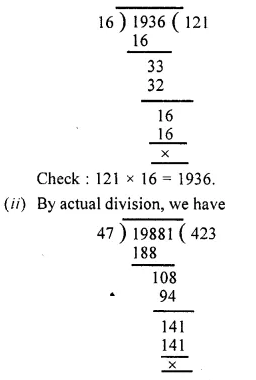Question 2.
Solution:
(i) By actual division, we have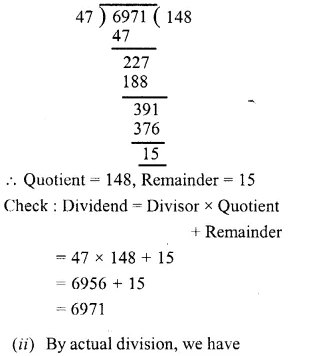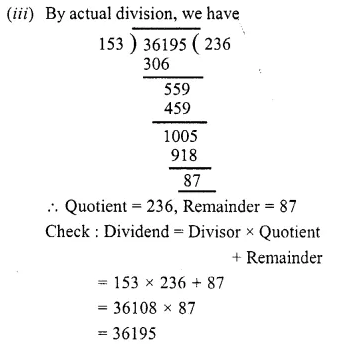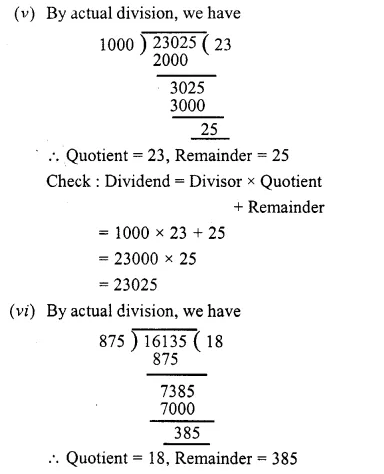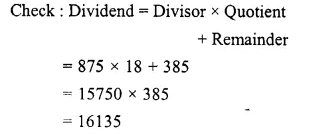Question 3.
Solution:
(i) We know that any number (non-zero) divided by 1 gives the number itself
65007 ÷ 1 = 65007
(ii) We know that 0 divided by any natural number gives 0
0 ÷ 879 = 0
(iii) 981 + 5720 ÷ 10 = 981 + (5720 ÷ 10)
= 981 + 572
= 1553
(iv) 1507 – 625 ÷ 25 = 1507 – (625 ÷ 25)
= 1507 – 25
= 1482
(v) 32277 ÷ (648 – 39)
= 32277 ÷ 609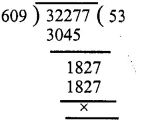32277 ÷ (648 – 39) = 53
(vi) 1573 ÷ 1573 – 1573 ÷ 1573
= (1573 ÷ 1573) – (1573 ÷ 1573)
= 1 – 1
= 0

Question 4.
Solution:
We have n ÷ n = n
let n = 1, 1 ÷ 1 = 1
1 = 1
which is true
∴ Hence 1 is the required whole number.

Question 5.
Solution:
Product of two numbers = 504347
One number = 317
Other number = 504347 ÷ 317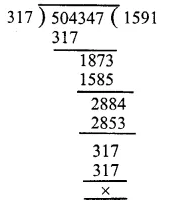∴ Other number = 1591

Question 6.
Solution:
Here Dividend = 59761, Quotient = 189
∴ Remainder = 37
We know that Dividend = Divisor x Quotient + Remainder
59761 = Divisor x 189 + 37
59761 – 37 = Divisor x 189
59724 = Divisor x 189
Divisor x 189 = 59724
∴ Divisor = 59724 ÷ 189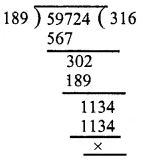∴ Divisor = 59724 ÷ 189 = 316

Question 7.
Solution:
Here dividend = 55390,
Divisor = 299 and Remainder = 75
By division algorithm, we have
Dividend = Quotient x Divisor + Remainder
55390 = Quotient x 299 + 75
55390 – 75 = Quotient x 299
55315 = Quotient x 299
Quotient x 299 = 55315
Quotient = 55315 ÷ 299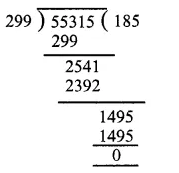∴ Required quotient = 185

Question 8.
Solution:
On dividing 13601 by 87, we haveIt is clear that if we subtract 29 from 13601, the resulting number will be exactly divisible by 87.
∴ The required least number = 29.

Question 9.
Solution:
Here dividend = 1056, Divisor = 23
By actual division, we have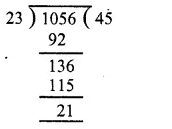It is clear that if we add 2 to 21, it will become 23 which is divisible by 23.
∴ Required least number = 2.

Question 10.
Solution:
Greatest 4-digit number = 9999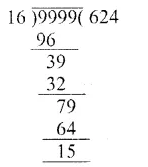On, dividing by 16, we get remainder as 15
∴ The required largest 4-digit number = 9999 – 15
= 9984

Question 11.
Solution:
Largest number of 5-digits = 99999
On dividing 99999 by 653, we have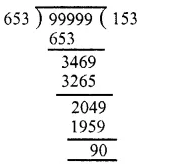∴ Quotient = 153, Remainder = 90
Check : By division algorithm Dividend = Divisor x Quotient + Remainder
= 653 x 153 + 90
= 99909 + 90
= 99999

Question 12.
Solution:
The least 6-digit number = 100000
On dividing 100000 by 83, we have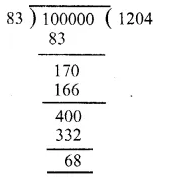It is clear that if we add 15 to 68, it will become 83 which is divisible by 83.
∴ Required least 6-digit number = 100000 + 15
= 100015

Question 13.
Solution:
Cost of 1 dozen bananas = Rs. 29
Bananas can be purchase in Rs. 13921392 ÷ 29
= 48 dozens

Question 14.
Solution:
Total number of trees = 19625
Total number of rows = 157
Number of trees in each row = 19625 ÷ 157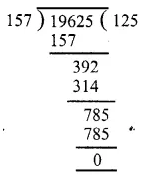∴ Number of trees in each row = 125

Question 15.
Solution:
Total population of the town = 517530
Since there is one educated person out of 15
Total number of educated persons in the town = 517530 ÷ 15∴ Total number of educated persons in the town = 34502.

Question 16.
Solution:
Cost of 23 colour TV sets = Rs. 570055
Cost of 1 colour TV set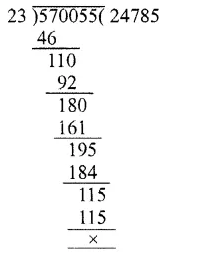∴ Cost of 1 color TV set
= Rs. 570055 ÷ 23
= Rs. 24785

Hope given RS Aggarwal Solutions Class 6 Chapter 3 Whole Numbers Ex 3E are helpful to complete your math homework.

If you have any doubts, please comment below. Learn Insta try to provide online math tutoring for you.